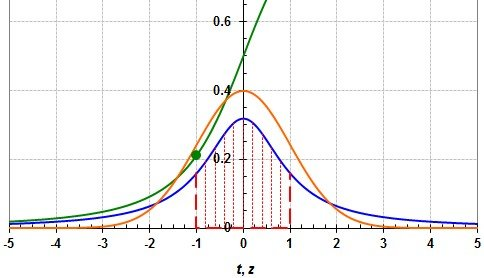# Student’s t Distribution

Student’s t distribution, or simply called t-distribution, is a form of continuous probability distributions which is formed when we are trying to estimate the mean of a population that is normally distributed, but we have a small sample size and we don’t know the population standard deviation. When we say that the sample size is small, we mean a sample size of less than 30.

The t-statistic is calculated as follows:

$t-statistic = \frac{\bar{x}-\mu }{\frac{s}{\sqrt{n}}}$

Where,

x-bar is the sample mean

µ is the population mean

s is the sample standard deviation

n is the sample size.

It’s the distribution of this t-statistic which is called t-distribution or Student-t distribution.

The t-distribution is symmetric and bell-shaped just like a normal distribution. However, it has heavier tails, which means more observations are far from the mean.

In the above formula, note that if the sample size was greater than 30, then it will be the z-statistic, because when the sample size is greater than 30, then the use of sample standard deviation for calculating the z-statistic is considered OK. This means that the z-statistic (approximation) will be normally distributed if our sample size is greater than 30. However, when n is less than 30, the statistic will not be normally distributed.There will be a different t-distribution for each sample size, so it will be a class of distributions.

The t-distribution has a degree of freedom equal to n-1. The larger the degree of freedom, the closer is the t-distribution to normal distribution (z-statistic).

Get smart about tech at work.

As a non-technical professional, learn how software works with simple explanations of tech concepts. Learn more...

# Data Science for Finance Bundle: 43% OFF

Get our Data Science for Finance Bundle for just $29$51.